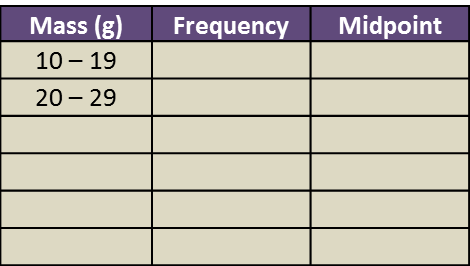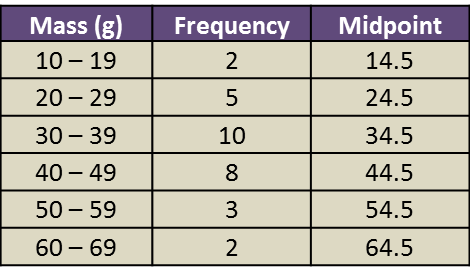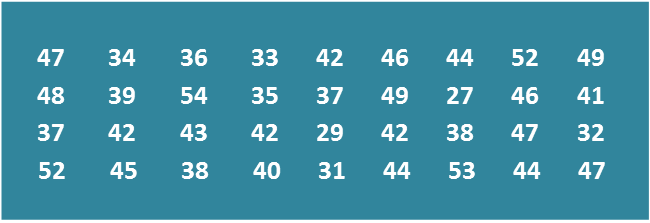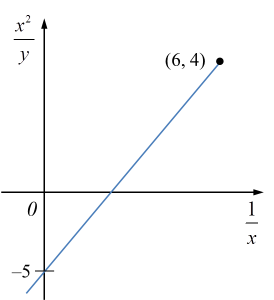# 6.7 SPM Practice (Long Questions)

Question 9 (12 marks):
The data in the Diagram shows the mass, in g, of 30 strawberries plucked by a tourist from a farm.Diagram

(a)
Based on the Diagram, complete Table 3 in the answer space.

(b)
Based on Table, calculate the estimated mean mass of a strawberry.

(c)
For this part of the question, use graph paper.
By using the scale of 2 cm to 10 g on the horizontal axis and 2 cm to 1 strawberry on the vertical axis, draw a histogram for the data.

(d)
Based on the histogram drawn in 14(c), state the number of strawberries with the mass of more than 50 g.Solution:
(a)(b)

(c)(d)
Number of strawberries with the mass more than 50 g
= 3 + 2
= 5

# 6.7 SPM Practice (Long Questions)

Question 8 (12 marks):
Diagram shows the marks obtained by a group of 36 students in a Mathematics test.Diagram

(a)
Based on the data in Diagram, complete Table in the answer space.

(b)
Based on the Table, calculate the estimated mean mark of a student.

(c)
For this part of the question, use graph paper.
By using the scale of 2 cm to 5 marks on the horizontal axis and 2 cm to 1 student on the vertical axis, draw a frequency polygon for the data.

(d)
Based on the frequency polygon in 8(c), state the number of students who obtained more than 40 marks.Table

Solution:
(a)(b)

(c)(d)
Number of students who obtained more than 40 marks
= 10 + 8 + 4
= 22 students

# SPM Practice Question 2

Question 2:
The third term and the sixth term of a geometric progression are 24 and $7\frac{1}{9}$ respectively. Find
(a) the first term and the common ratio,
(b) the sum of the first five terms,
(c) the sum of the first n terms with n is very big approaching rn ≈ 0.

Solution:
(a)

(b)

(c)

Therefore, sum of the first n terms with n is very big approaching rn ≈ 0 is 162.

# Short Questions (Question 7 & 8)

Question 7:
The masses of mangoes in a stall have a normal distribution with a mean of 200 g and a standard deviation of 30 g.
(a) Find the mass, in g, of a mango whose z-score is 0.5.
(b) If a mango is chosen at random, find the probability that the mango has a mass of at least 194 g.

Solution:
µ = 200 g
σ = 30 g
Let X be the mass of a mango.

(a)
$\begin{array}{l}\frac{X-200}{30}=0.5\\ X=0.5\left(30\right)+200\\ X=215g\end{array}$

(b)
$\begin{array}{l}P\left(X\ge 194\right)\\ =P\left(Z\ge \frac{194-200}{30}\right)\\ =P\left(Z\ge -0.2\right)\\ =1-P\left(Z>0.2\right)\\ =1-0.4207\\ =0.5793\end{array}$

Question 8:
Diagram below shows a standard normal distribution graph.The probability represented by the area of the shaded region is 0.3238.
(a) Find the value of k.
(b) X is a continuous random variable which is normally distributed with a mean of 80 and variance of 9.
Find the value of X when the z-score is k.

Solution:
(a)
P(Z > k) = 0.5 – 0.3238
= 0.1762
k = 0.93

(b)
µ = 80,
σ2 = 9, σ = 3
$\begin{array}{l}\frac{X-80}{3}=0.93\\ X=3\left(0.93\right)+80\\ X=82.79\end{array}$

# Short Questions (Question 5 & 6)

Question 5:
Diagram below shows the graph of a binomial distribution of X.

(a) the value of h,
(b) P (X ≥ 3)

Solution:
(a)
P (X = 0) + P (X = 1) + P (X = 2) + P (X = 3) + P (X = 4) = 1
$\begin{array}{l}\frac{1}{16}+\frac{1}{4}+h+\frac{1}{4}+\frac{1}{16}=1\\ h=1-\frac{5}{8}\\ h=\frac{3}{8}\end{array}$

(b)
P (X ≥ 3) = P (X = 3) + P (X = 4)
$P\left(X\ge 3\right)=\frac{1}{4}+\frac{1}{16}=\frac{5}{16}$

Question 6:
The random variable X represents a binomial distribution with 10 trails and the probability of success is ¼.
(a) the standard deviation of the distribution,
(b) the probability that at least one trial is success.

Solution:
(a)
n = 10, p = ¼
$\begin{array}{l}\text{Standard deviation}=\sqrt{npq}\\ \text{}=\sqrt{10×\frac{1}{4}×\frac{3}{4}}\\ \text{}=1.875\end{array}$

(b)
$\begin{array}{l}P\left(X=r\right)=C{}_{r}{\left(\frac{1}{4}\right)}^{r}{\left(\frac{3}{4}\right)}^{10-r}\\ P\left(X\ge 1\right)\\ =1-P\left(X<1\right)\\ =1-P\left(X=0\right)\\ =1-C{}_{0}{\left(\frac{1}{4}\right)}^{0}{\left(\frac{3}{4}\right)}^{10}\\ =0.9437\end{array}$

# SPM Practice 2 (Question 11 & 12)

Question 11 (3 marks):
Diagram 6 shows the graph of a straight lineDiagram 11

Based on Diagram 6, express y in terms of x.

Solution:

Question 12 (3 marks):
The variables x and y are related by the equation $y=x+\frac{r}{{x}^{2}}$ , where r is a constant. Diagram 8 shows a straight line graph obtained by plottingDiagram 12

Express h in terms of p and r.

Solution:

# SPM Practice 2 (Linear Law) – Question 1

Question 1 (10 marks):
Use a graph to answer this question.
Table 1 shows the values of two variables, x and y, obtained from an experiment. A straight line will be obtained when a graph of is plotted.(a) Based on Table 1, construct a table for the values of

(c) Using the graph in 1(b)
(i) find the value of y when x = 2.7,
(ii) express y in terms of x.

Solution:
(a)(b)(c)(i)

(c)(ii)

# SPM Practice 3 (Linear Law) – Question 6

Question 6
The table below shows the values of two variables, x and y, obtained from an experiment. The variables x and y are related by the equation $\frac{a}{y}=\frac{b}{x}+1$ , where k and p are constants.

(a) Based on the table above, construct a table for the values of $\frac{1}{x}$ and $\frac{1}{y}$ . Plot $\frac{1}{y}$ against $\frac{1}{x}$ , using a scale of  2 cm to 0.1 unit on the $\frac{1}{x}$ - axis and  2 cm to 0.2 unit on the $\frac{1}{y}$ - axis. Hence, draw the line of best fit.
(b) Use the graph from  (b)  to find the value of
(i)  a,
(ii)  b.

Solution

Step 1 : Construct a table consisting X and Y.

Step 2 : Plot a graph of Y against X, using the scale given and draw a line of best fit

Step 3 : Calculate the gradient, m, and the Y-intercept, c, from the graph

Step 4 : Rewrite the original equation given and reduce it to linear form

Step 5 : Compare with the values of m and c obtained, find the values of the unknown required

# SPM Practice 3 (Linear Law) – Question 5

Question 5
The following table shows the corresponding values of two variables, x and y, that are related by the equation $y=p{k}^{\sqrt{x}}$ , where p and k are constants.

(a) Plot $log 10 y$ against $x$  .  Hence, draw the line of best fit

(b) Use your graph in (a) to find the values of p and k.

# SPM Practice 2 (Linear Law) – Question 4

Question 4
The table below shows the corresponding values of two variables, x and y, that are related by the equation $y=qx+\frac{p}{qx}$ , where p and q are constants.

One of the values of y is incorrectly recorded.
(a) Using scale of 2 cm to 5 units on the both axis, plot the graph of xy against ${x}^{2}$  .  Hence, draw the line of best fit

(i) State the values of y which is incorrectly recorded and determine its actual value.
(ii) Find the value of p and of q.

Solution
Step 1 : Construct a table consisting X and Y.

Step 2 : Plot a graph of Y against X, using the scale given and draw a line of best fit